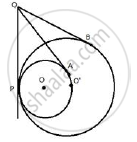Share

# Two Circle Touch Each Other Internally. Show that the Tangents Drawn to the Two Circles from Any Point on the Common Tangent Are Equal in Length. - Mathematics

Course

#### Question

Two circle touch each other internally. Show that the tangents drawn to the two circles from any point on the common tangent are equal in length.

#### SolutionFrom Q, QA and QP are two tangents to the circle with centre O
Therefore, QA = QP .......(i)
Similarly, from Q, QB and QP are two tangents to the circle with centre O'
Therefore, QB = QP .......(ii)
From (i) and (ii)
QA = QB
Therefore, tangents QA and QB are equal.

Is there an error in this question or solution?